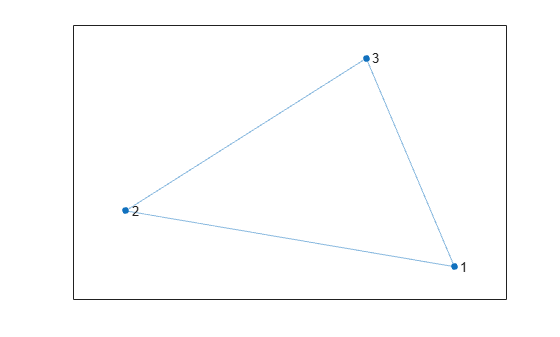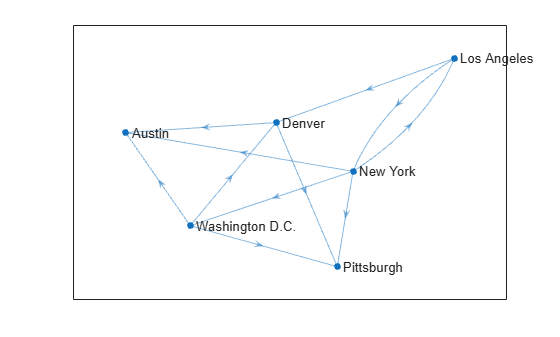# rmnode

Remove node from graph

## Syntax

``H = rmnode(G,nodeIDs)``

## Description

example

````H = rmnode(G,nodeIDs)` removes the nodes specified by `nodeIDs` from graph `G`. Any edges incident upon the nodes in `nodeIDs` are also removed. `rmnode` refreshes the numbering of the nodes in `H`, such that if you removed node `k`, then nodes `1:k-1` have the same node numbers in `H`, and nodes `k+1:numnodes(G)` in `G` become `k:numnodes(H)` in `H`.```

## Examples

collapse all

Create and plot a graph.

```s = [1 1 1 2 2 3]; t = [2 3 4 3 4 4]; G = graph(s,t); plot(G)```Remove node 1 from the graph and plot the result. The nodes in the new graph are automatically renumbered.

```G = rmnode(G,1); plot(G)```Create and plot a graph with named nodes.

```s = [1 1 1 1 2 2 3 3 3 5 5]; t = [2 3 4 6 1 5 4 5 6 4 6]; names = {'New York' 'Los Angeles' 'Washington D.C.' 'Pittsburgh' ... 'Denver' 'Austin'}; G = digraph(s,t,[],names); plot(G)```Remove the nodes `'New York'` and `'Pittsburgh'` from the graph, then replot the result.

```G = rmnode(G,{'New York' 'Pittsburgh'}); plot(G,'Layout','force')```## Input Arguments

collapse all

Input graph, specified as either a `graph` or `digraph` object. Use `graph` to create an undirected graph or `digraph` to create a directed graph.

Example: `G = graph(1,2)`

Example: `G = digraph([1 2],[2 3])`

Node identifiers, specified as one or more node indices or node names.

This table shows the different ways to refer to one or more nodes either by their numeric node indices or by their node names.

FormSingle NodeMultiple Nodes
Node index

Scalar

Example: `1`

Vector

Example: `[1 2 3]`

Node name

Character vector

Example: `'A'`

Cell array of character vectors

Example: `{'A' 'B' 'C'}`

String scalar

Example: `"A"`

String array

Example: `["A" "B" "C"]`

Example: `G = rmnode(G,[1 2])` removes node 1 and node 2 from graph `G`.

## Output Arguments

collapse all

Output graph, returned as a `graph` or `digraph` object.